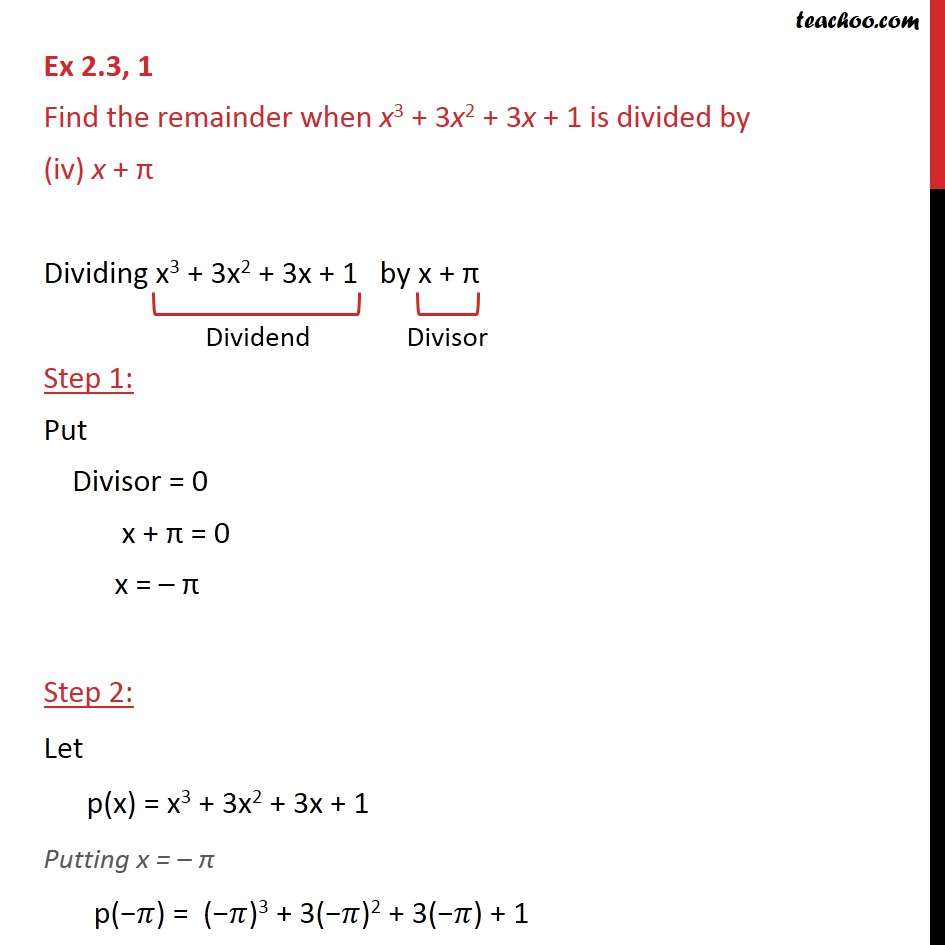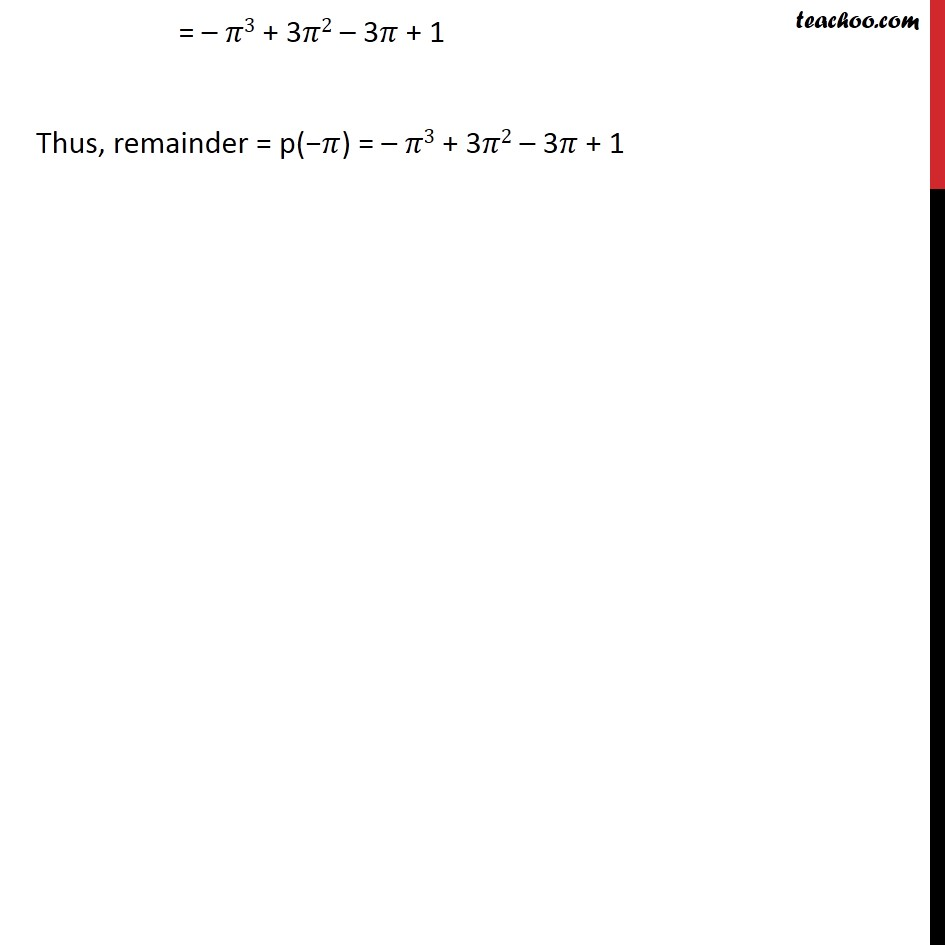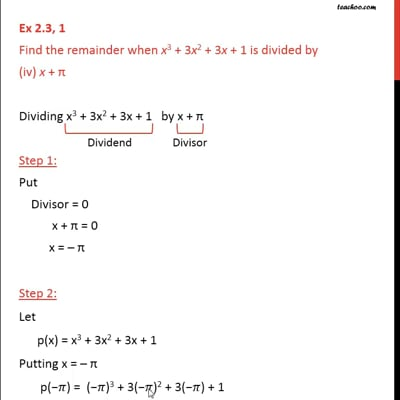Ex 2.3

Chapter 2 Class 9 Polynomials
Serial order wiseThis video is only available for Teachoo black users

Maths Crash Course - Live lectures + all videos + Real time Doubt solving!

### Transcript

Ex 2.3, 1 Find the remainder when x3 + 3x2 + 3x + 1 is divided by (iv) x + π Dividing x3 + 3x2 + 3x + 1 by x + π Step 1: Put Divisor = 0 x + π = 0 x = – π Step 2: Let p(x) = x3 + 3x2 + 3x + 1 Putting x = – π p("−" 𝜋) = ("−" 𝜋)3 + 3("−" 𝜋)2 + 3("−" 𝜋) + 1 = – 𝜋3 + 3𝜋2 – 3𝜋 + 1 Thus, remainder = p("−" 𝜋) = – 𝜋3 + 3𝜋2 – 3𝜋 + 1